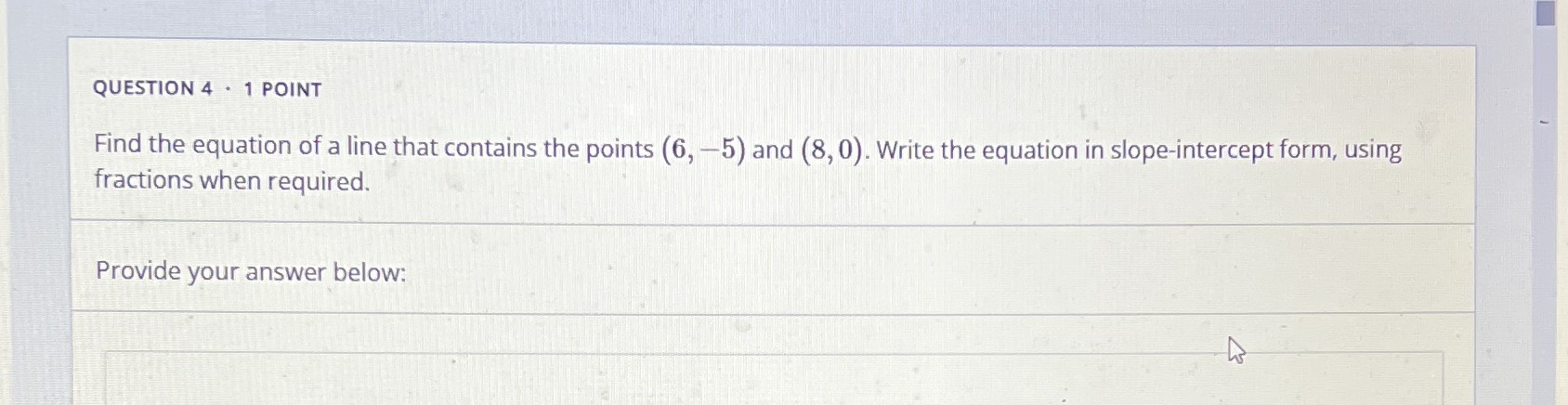### ¿Todavía tienes preguntas de matemáticas?

Pregunte a nuestros tutores expertos
Algebra
Pregunta(6,-5) and (8,0)write the equation in slope intercept form using fractions when required.

QUESTION $$4$$ . 1 POINT Find the equation of a line that contains the points $$( 6 , - 5 )$$ and $$( 8,0 )$$ . Write the equation in slope-intercept form, using fractions when required. Provide your answer below: